### Home > INT3 > Chapter Ch8 > Lesson 8.1.3 > Problem8-50

8-50.
1. For each of the following polynomial functions, state the degree, list the coefficients, and then label them a0 through an. Refer to the example in the Math Notes box in Lesson 8.1.1 about polynomial notation. Homework Help ✎

1. P(x) = 6x4 – 3x3 + 5x2 + x + 8

2. P(x) = –5x3 + 10x2 + 8

3. P(x) = –x2 + x

4. P(x) = x(x – 3)(x – 5)

5. P(x) = x

6. P(x) = 10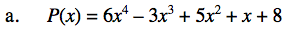Degree 4,
a4 = 6, a3 = −3, a2 = 5,
a1 = 1, a0 = 8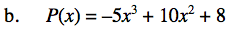Degree 3,
a3 = −5, a2 = 10,
a1 = 0, a0 = 8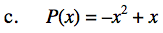See the Math Notes box and parts (a) and (b).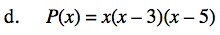Write the polynomial in standard form first.

Degree 3,
a3 = 1, a2 = −8,
a1 = 15, a0 = 0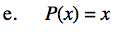See the Math Notes box and parts (a) and (b).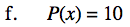See the Math Notes box and parts (a) and (b).